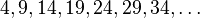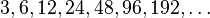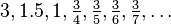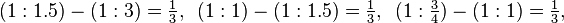# Sequence facts for kids

Kids Encyclopedia Facts

A sequence is a word meaning "coming after or next, a series".

It is used in mathematics and other disciplines. In ordinary use it means a series of events, one following another. In maths, a sequence is made up of several things put together, one after the other. The order that the things are in matters: (Blue, Red, Yellow) is a sequence, and (Yellow, Blue, Red) is a sequence, but they are not the same. Sequences made up of numbers are also called progressions.

There are two kinds of sequences. One kind is finite sequences, which have an end. For example, (1, 2, 3, 4, 5) is a finite sequence. Sequences can also be infinite, which means they keep going and never end. An example of a sequence that is infinite is the sequence of all even numbers, bigger than 0. This sequence never ends: it starts with 2, 4, 6, and so on, and you can always keep on naming even numbers.

If a sequence is finite, it is easy to say what it is: you can just write down all the things in the sequence. This does not work for an infinite sequence. So another way to write down a sequence is to write a rule for finding the thing in any place you want. The rule should tell us how to get the thing in the n-th place, if n can be any number. If you know what a function is, this means that a sequence is a kind of function.

For example, the rule could be that the thing in the n-th place is the number 2×n (2 times n). This tells us what the whole sequence is, even though it never ends. The first number is 2×1, which is 2. The second number is 2×2, or 4. If we want to know the 100-th number, it's 2×100, or 200. No matter which thing in the sequence we want, the rule can tell us what it is.

## Types of sequences

### Arithmetic progressions (AP)

The difference between a term and the term before it, is always a constant.

Example:$4, 9, 14, 19, 24, 29, 34, \ldots$

9 - 4 = 5, 14 - 9 = 5, 19 - 14 = 5, 24 - 19 = 5, and so on

so if you take the first term as A and the constant difference as D the general formula for arithmetic sequence is T=a+(n-1)D where n is the number of term

### Geometric progressions (GP)

The ratio between a term and the term before it, is always constant.

Example:$3, 6, 12, 24, 48, 96, 192, \ldots$

6 : 3 = 2, 12 : 6 = 2, 24 : 12 = 2, 48 : 24 = 2, and so on

the general formula is T=ar^(n-1) where a is the first term , r is the ratio and n is the number of term.

### Harmonic Progressions (HP)

The difference between the reciprocal of a term and the reciprocal of the term before it, is a constant.

Example:$3, 1.5, 1, \tfrac34, \tfrac35, \tfrac36, \tfrac37, \ldots$$(1 : 1.5) - (1 : 3) = \tfrac13, \, \, \, (1 : 1) - (1 : 1.5) = \tfrac13, \, \, \, (1 : \tfrac34) - (1 : 1) = \tfrac13,$ and so on

## Series

A series is the sum of all the terms of a sequence.

general formula for calculating sum of arithmetic sequence is

S=n/2 [2a=(n-1)d]

that of geometric sequence is

S= a/(1-r) if the sequence is infinite and S= [a(1-r^n)]/(1-r) if it is finite

here a is the first term , d is the common difference in arithmetic sequence , r is the ratio n geometric sequence and n is the number of term.Sequence Facts for Kids. Kiddle Encyclopedia.